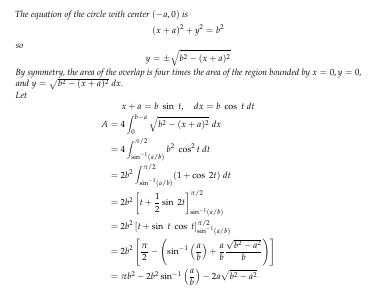# How to Simplify Sympy Symbolic Integration

Hi all,

I have two circles of radius b, their centers 2a apart (0 \le a \le b). The question is, why is the SymPy computation resulting in a very complex formula?
While in the solution manual it can be explained easily resulting in one line of answer.

The code:

``````using SymPy
@syms x, a, b

v1(x) = sqrt(b^2 - (x+a)^2)

V1 = integrate((v1(x)), (x,0,b-a))

println("Computing symbolic integral")
println("  ", 4*simplify(V1))

``````